# HSSlive: Plus One & Plus Two Notes & Solutions for Kerala State Board

## BSEB Class 10 Maths Chapter 14 Statistics Ex 14.1 Textbook Solutions PDF: Download Bihar Board STD 10th Maths Chapter 14 Statistics Ex 14.1 Book AnswersBSEB Class 10 Maths Chapter 14 Statistics Ex 14.1 Textbook Solutions PDF: Download Bihar Board STD 10th Maths Chapter 14 Statistics Ex 14.1 Book Answers

BSEB Class 10th Maths Chapter 14 Statistics Ex 14.1 Textbooks Solutions and answers for students are now available in pdf format. Bihar Board Class 10th Maths Chapter 14 Statistics Ex 14.1 Book answers and solutions are one of the most important study materials for any student. The Bihar Board Class 10th Maths Chapter 14 Statistics Ex 14.1 books are published by the Bihar Board Publishers. These Bihar Board Class 10th Maths Chapter 14 Statistics Ex 14.1 textbooks are prepared by a group of expert faculty members. Students can download these BSEB STD 10th Maths Chapter 14 Statistics Ex 14.1 book solutions pdf online from this page.

## Bihar Board Class 10th Maths Chapter 14 Statistics Ex 14.1 Books Solutions

 Board BSEB Materials Textbook Solutions/Guide Format DOC/PDF Class 10th Subject Maths Chapter 14 Statistics Ex 14.1 Chapters All Provider Hsslive

## How to download Bihar Board Class 10th Maths Chapter 14 Statistics Ex 14.1 Textbook Solutions Answers PDF Online?

2. Click on the Bihar Board Class 10th Maths Chapter 14 Statistics Ex 14.1 Answers.
3. Look for your Bihar Board STD 10th Maths Chapter 14 Statistics Ex 14.1 Textbooks PDF.
4. Now download or read the Bihar Board Class 10th Maths Chapter 14 Statistics Ex 14.1 Textbook Solutions for PDF Free.

Find below the list of all BSEB Class 10th Maths Chapter 14 Statistics Ex 14.1 Textbook Solutions for PDF’s for you to download and prepare for the upcoming exams:

## BSEB Bihar Board Class 10th Maths Solutions Chapter 14 Statistics Ex 14.1

Question 1.
A survey was conducted by a group of students as a part of their environment awareness programme, in which they collected the following data regarding the number of plants in 20 houses in a locality. Find the mean number of plants per house.Which method did you use for finding the mean, and why?
Solution:
Calculation of MeanWe have used direct method because numerical values of xi and fi are small.

Question 2.
Consider the following distribution of daily wages of 50 workers of a factory :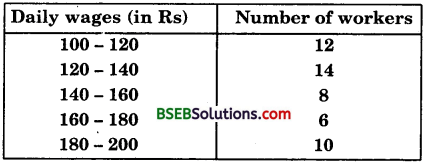Find the mean daily wages of the workers of the factory by using an appropriate method.
Solution:
Let the assumed mean, A = 150 and class-size, h = 20.
So, ui = 𝑥𝑖−Aℎ = 𝑥𝑖−15020
We construct the table :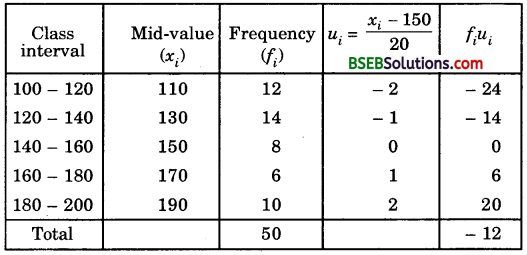𝐸¯ = A + hΣ𝑓𝑖𝑢𝑖Σ𝑓𝑖 = 150 + 20 x −1250
= 150 – 4.8
= 145.2
Hence, mean = Rs 145.20

Question 3.
The following distribution shows the daily pocket allowdnce of children of a locality. The mean pocket allowance is Rs 18. Find the missing frequency f.Solution:
Let the assumed mean, A = 16, class size, h = 2,
So, ui = 𝑥𝑖−Aℎ = 𝑥𝑖−162We have : 𝑥¯ = 18, A = 16 and h – 2.Hence, the missing frequency is 20.

Question 4.
Thirty women were examined in a hospital by a doctor and the number of heart beats per minute were recorded and summarised as follows. Find the mean heart beats per minute for these women, choosing a suitable method.Solution:
Let the assumed mean, A = 75.5 and class size, h = 3.
So, ui = 𝑥𝑖−Aℎ = 𝑥𝑖−75.53
We construct the following table :So, 𝑥¯ = A + hΣ𝑓𝑖𝑢𝑖Σ𝑓𝑖 = 75.6 + 3 x 430
= 75.5 + 0.4 = 75.9
Hence, the mean heart beats per minute for these women is 75.9.

Question 5.
In a retail market, fruit vendors were selling mangoes kept in packing boxes. These boxes contained varying number of mangoes. The following was the distribution of mangoes according to the number of boxes.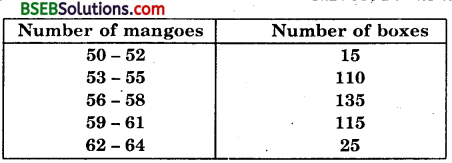Find the mean number of mangoes kept in a packing box. Which method of finding the mean did you choose?
Solution:
Here, the class intervals are formed by the exclusive method. If we make the data an inclusive one, the mid-values remain same. So, there is no need to convert the data with the caution that while finding h, we should count both the limits of class interval. For example, for 53 – 55, both 53 and 55 should be counted and thus h – 3 and not 55 – 53 = 2.
Let the assumed mean be A = 60 and h = 3.
So, ui = 𝑥𝑖−Aℎ = 𝑥𝑖−603
Calculation of Mean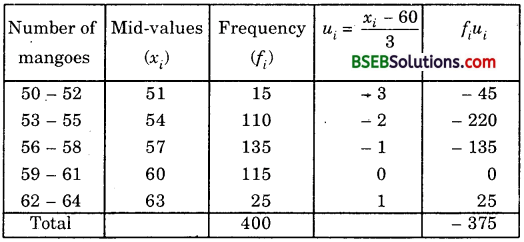∴ 𝑥¯ = A + hΣ𝑓𝑖𝑢𝑖Σ𝑓𝑖 = 60 + 3 x −375400
= 60 – 2.8125 = 57.1875 = 57.19 (nearly)
Hence, mean number of mangoes per box is 57.19.
Here, we have used the step-deviation method for finding the mean.

Question 6.
The table below shows the daily expenditure on food of 25 households in a locality.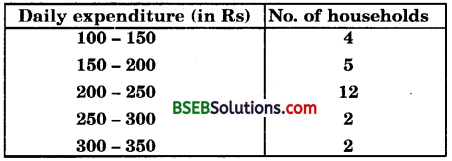Find the mean daily expenditure on food by a suitable method.
Solution:
Let the assumed mean, A = 225, class size, h = 50
So, ui = 𝑥𝑖−Aℎ = 𝑥𝑖−22550
We construct the following table :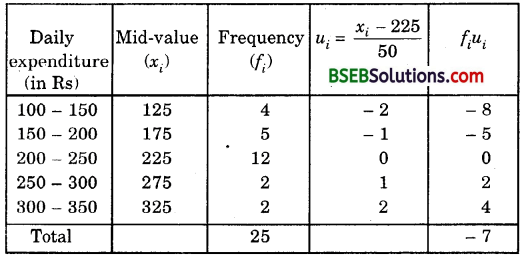So, 𝑥¯ = A + hΣ𝑓𝑖𝑢𝑖Σ𝑓𝑖
= 225 + 50 x −725
= 225 – 14 = 211
Hence, the mean daily expenditure on food is Rs 211.

Question 7.
To find the concentration of SO2 in the air (in parts per million, i.e., ppm), the data was collected for 30 localities in a certain city and is presented below :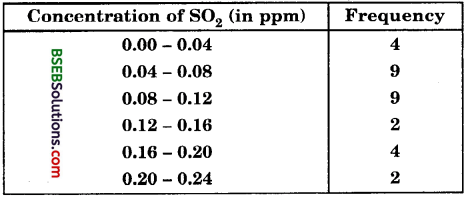Find the mean concentration of SO2 in the air.
Solution:
Calculation of mean by direct methodQuestion 8.
A class teacher has the following absentee record of 40 students of a class for the whole term. Find the mean number of days a student was absent.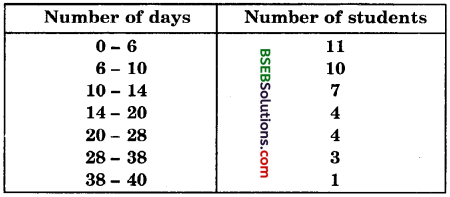Solution:
Here, the class size varies, and xi’s are small.
Let us apply the direct method here :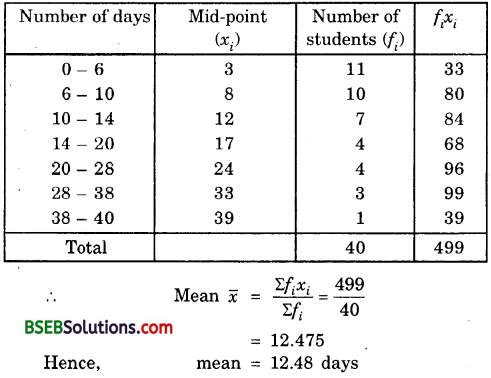Question 9.
The following table gives the literacy rate (in percentage) of 35 cities. Find the mean literacy rate.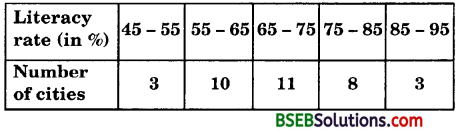Solution:
Let the assumed mean, A = 70 and class size, h = 10.
So, ui = 𝑥𝑖−Aℎ = 𝑥𝑖−7010Thus, mean literacy rate is 69.43%.

## Bihar Board Class 10th Maths Chapter 14 Statistics Ex 14.1 Textbooks for Exam Preparations

Bihar Board Class 10th Maths Chapter 14 Statistics Ex 14.1 Textbook Solutions can be of great help in your Bihar Board Class 10th Maths Chapter 14 Statistics Ex 14.1 exam preparation. The BSEB STD 10th Maths Chapter 14 Statistics Ex 14.1 Textbooks study material, used with the English medium textbooks, can help you complete the entire Class 10th Maths Chapter 14 Statistics Ex 14.1 Books State Board syllabus with maximum efficiency.

## FAQs Regarding Bihar Board Class 10th Maths Chapter 14 Statistics Ex 14.1 Textbook Solutions

#### Can we get a Bihar Board Book PDF for all Classes?

Yes you can get Bihar Board Text Book PDF for all classes using the links provided in the above article.

## Important Terms

Bihar Board Class 10th Maths Chapter 14 Statistics Ex 14.1, BSEB Class 10th Maths Chapter 14 Statistics Ex 14.1 Textbooks, Bihar Board Class 10th Maths Chapter 14 Statistics Ex 14.1, Bihar Board Class 10th Maths Chapter 14 Statistics Ex 14.1 Textbook solutions, BSEB Class 10th Maths Chapter 14 Statistics Ex 14.1 Textbooks Solutions, Bihar Board STD 10th Maths Chapter 14 Statistics Ex 14.1, BSEB STD 10th Maths Chapter 14 Statistics Ex 14.1 Textbooks, Bihar Board STD 10th Maths Chapter 14 Statistics Ex 14.1, Bihar Board STD 10th Maths Chapter 14 Statistics Ex 14.1 Textbook solutions, BSEB STD 10th Maths Chapter 14 Statistics Ex 14.1 Textbooks Solutions,
Share: